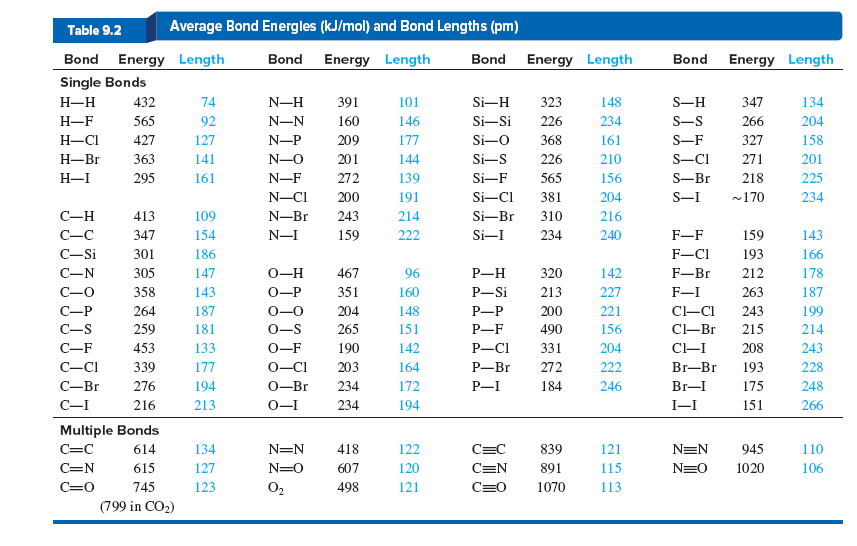# Problem: There are two main types of covalent bond breakage. In homolytic breakage (as in following Table), each atom in the bond gets one of the shared electrons. In some cases, the electronegativity of adjacent atoms affects the bond energy. In heterolytic breakage, one atom gets both electrons and the other gets none; thus, a cationand an anion form.(b) Use bond energy and any other data to calculate the enthalpy of reaction for the heterolytic cleavage of O2.

95% (64 ratings)
###### Problem Details

There are two main types of covalent bond breakage. In homolytic breakage (as in following Table), each atom in the bond gets one of the shared electrons. In some cases, the electronegativity of adjacent atoms affects the bond energy. In heterolytic breakage, one atom gets both electrons and the other gets none; thus, a cationand an anion form.

(b) Use bond energy and any other data to calculate the enthalpy of reaction for the heterolytic cleavage of O2.Frequently Asked Questions

What scientific concept do you need to know in order to solve this problem?

Our tutors have indicated that to solve this problem you will need to apply the Bond Energy concept. You can view video lessons to learn Bond Energy. Or if you need more Bond Energy practice, you can also practice Bond Energy practice problems.

What textbook is this problem found in?

Our data indicates that this problem or a close variation was asked in Chemistry: The Molecular Nature of Matter and Change - Silberberg 8th Edition. You can also practice Chemistry: The Molecular Nature of Matter and Change - Silberberg 8th Edition practice problems.